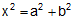Problem 367. Square, Angle, 45 degrees, Pythagoras, Congruence, Similarity. Level: High School, College, SAT Prep. In the figure ABCD is a square and the measure of angle EAF is 45 degrees. If BG = a, DH = b and GH = x, prove that.Recent Additions